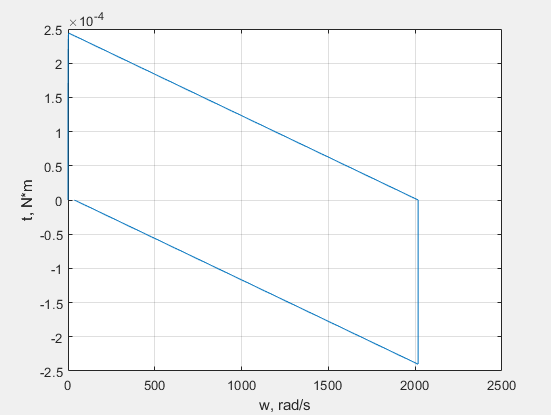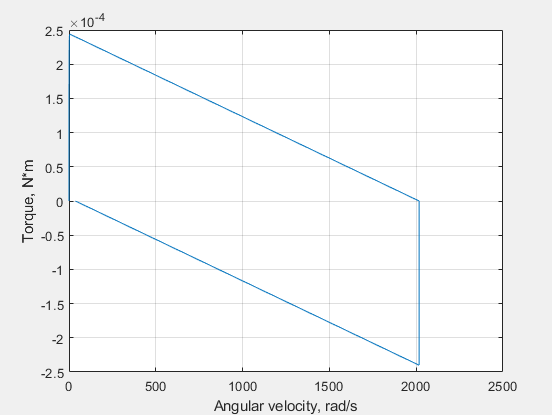# plotxy

Plot series associated with two node objects against each other

## Syntax

``fh = plotxy(x,y)``
``fh = plotxy(x,y,Name,Value)``

## Description

example

````fh = plotxy(x,y)` plots the simulation series values of node `y` along the `y`-axis, with series values of node `x` along the `x`-axis. `fh` is a cell array of handles to the resulting figures. `x` and `y`are `simscape.logging.Node` objects or homogeneous cell arrays of such objects. Each object must be a simulation variable node (one that has a direct child series). The values of this child series are plotted along the respective axis. All series must have the same time vectors. If `x` and `y` are cell arrays, they must be of the same size, or one of them can be a scalar. ```

example

````fh = plotxy(x,y,Name,Value)` lets you customize the plot by using one or more `Name,Value` pair arguments. For example, specify '`time`' followed by a 1x2 vector, ```[start_time end_time]```, to plot only the data within this time range.```

## Examples

collapse all

Plot the motor torque variable node against its angular velocity variable node.

Open the Permanent Magnet DC Motor example model:

```openExample('simscape/PermanentMagnetDCMotorExample') ```

This example model has data logging enabled for the whole model, with the Workspace variable name parameter set to `simlog_PermanentMagnetDCMotor`.

Simulate the model to log the simulation data:

`sim('PermanentMagnetDCMotor');`

Plot the motor torque against its angular velocity:

```simscape.logging.plotxy(simlog_PermanentMagnetDCMotor.DC_Motor.Rotational_Electromechanical_Converter.R.w,... simlog_PermanentMagnetDCMotor.DC_Motor.Inertia.t)```When you plot the variable nodes against each other, the default plot displays the name of the variable and the unit name along each axis. To customize the axis names, use name-value pair arguments.

```simscape.logging.plotxy(simlog_PermanentMagnetDCMotor.DC_Motor.Rotational_Electromechanical_Converter.R.w,... simlog_PermanentMagnetDCMotor.DC_Motor.Inertia.t,'xname','Angular velocity','yname','Torque')```## Input Arguments

collapse all

Simulation data to plot along the x-axis, specified as a `simscape.logging.Node` object or a homogeneous cell array of such objects. Each object must be a simulation variable node (one that has a direct child series). The values of this child series are plotted along the respective axis. If `x` and `y` are cell arrays, they must be of the same size, or one of them can be a scalar. All series must have the same time vectors. `x` must include a full identifier path to the node, starting with the workspace log variable name.

Simulation data to plot along the y-axis, specified as a `simscape.logging.Node` object or a homogeneous cell array of such objects. Each object must be a simulation variable node (one that has a direct child series). The values of this child series are plotted along the respective axis. If `x` and `y` are cell arrays, they must be of the same size, or one of them can be a scalar. All series must have the same time vectors. `y` must include a full identifier path to the node, starting with the workspace log variable name.

### Name-Value Arguments

Specify optional pairs of arguments as `Name1=Value1,...,NameN=ValueN`, where `Name` is the argument name and `Value` is the corresponding value. Name-value arguments must appear after other arguments, but the order of the pairs does not matter.

Before R2021a, use commas to separate each name and value, and enclose `Name` in quotes.

Example: ```fh = plotxy(simlog.TS.C.v,simlog.TS.R.v,'xunit','mm/s','yunit','mm/s')``` plots velocities of ports C and R of the Translational Spring block `TS` against each other, in `mm/s`.

Name of the plot x-axis, specified as the comma-separated pair consisting of '`xname`' and a character vector, string scalar, or a cell array of character vectors or string scalars. The number of elements in the cell array must be same as the number of elements of `x`.

Name of the plot y-axis, specified as the comma-separated pair consisting of '`yname`' and a character vector, string scalar, or a cell array of character vectors or string scalars. The number of elements in the cell array must be same as the number of elements of `y`.

Unit for plotting the data along the x-axis, specified as the comma-separated pair consisting of '`xunit`' and a unit name, or a cell array of unit names. Unit names must appear inside single quotes (`''`) or double quotes (`""`). Specified units must be commensurate with the units of the series values.

Unit for plotting the data along the y-axis, specified as the comma-separated pair consisting of '`xunit`' and a unit name, or a cell array of unit names. Unit names must appear inside single quotes (`''`) or double quotes (`""`). Specified units must be commensurate with the units of the series values.

## Output Arguments

collapse all

Handles to the resulting plot figure windows, one for each `y` versus `x` plot generated, returned as a cell array.

## Version History

Introduced in R2010b